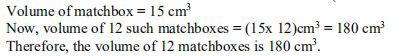Ncert solutionsOur top 5% students will be awarded a special scholarship to Lido.
Set your child up for success with Lido, book a class today!

# Question 1

A matchbox measures 4 cm x 2.5 cm x 1.5 cm.What will be the volume of a packet

containing 12 such boxes?

• Solution

• Transcript

Dimensions of a matchbox ( a cuboid) are l x b x h = 4 cm x 2.5 cm x 1.5 cm respectively

Formula to find the volume of matchbox = l x b x h = (4 x 2.5 x 1.5) = 15"hello students welcome to lido qna video session i am saf your math tutor and question for today is a match box measures 4 centimeter by 2.5 centimeter by 1.5 centimeter what will be the volume of the packet containing 12 such boxes so data given to us is the length breadth and height of the matchbox that is length is 4 centimeter breadth of the matchbox that is b 2.5 centimeter and height that is h that is equal to 1.5 centimeter now to find the volume we have the volume of the cuboid as matchbox is the cuboidal in shape we should take volume of the cuboid length into breath into height so 4 into 2.5 into 1.5 that is centimeter cube this is the volume of one match box now it is as that what will be the volume of twelve such boxes so volume of 12 such boxes you just multiply 15 into 12 and you will get 15 into 12 that is 180 centimeter cube that is your required volume of 12 match boxes therefore volume of 12 match boxes is 180 centimeter cube this is our final answer if you have any query you can drop it in our comment section and subscribe to lido for more such q a thank you for watching "

Our top 5% students will be awarded a special scholarship to Lido.Lido

Courses

Teachers

Book a Demo with us

Syllabus

Science
English
Coding

Terms & Policies

NCERT Question Bank

Maths
Science

Selina Question Bank

Maths
Physics
Biology

Allied Question Bank

Chemistry